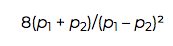Over a million developers have joined DZone.{{announcement.body}}
{{announcement.title}}

# Biased Random Number Generation

DZone 's Guide to

# Biased Random Number Generation

### When learning how to code or brushing up your skills, random number generators are a fun project. In this post, we go into some of the math behind these basic apps.

· Big Data Zone ·
Free Resource

Comment (0)

Save
{{ articles.views | formatCount}} Views

Melissa O'Neill has a new post on generating random numbers from a given range. She gives the example of wanting to pick a card from a deck of 52 by first generating a 32-bit random integer, then taking the remainder when dividing by 52. There's a slight bias because 232 is not a multiple of 52.

Since 232 = 82595524*52 + 48, there are 82595525 ways to generate the numbers 0 through 47, but only 82595524 ways to generate the numbers 48 through 51. As Melissa points out in her post, the bias here is small, but the bias increases linearly with the size of our "deck." To clarify, it is the relative bias that increases, not the absolute bias.

Suppose you want to generate a number between 0 and M, where M is less than 232 and not a power of 2. There will be 1 + ⌊232/ M⌋ ways to generate a 0, but ⌊232/ M⌋ ways to generate M-1. The difference in the probability of generating 0 vs generating M-1 is 1/232, independent of M. However, the ratio of the two probabilities is 1 + 1/⌊232/ M⌋ or approximately 1 + M/232.

As M increases, both the favored and unfavored outcomes become increasingly rare, but the ratio of their respective probabilities approaches 2.

Whether this makes any practical difference depends on your context. In general, the need for random number generator quality increases with the volume of random numbers needed.

Under conventional assumptions, the sample size necessary to detect a difference between two very small probabilities p1 and p2 is approximatelyand so, in the card example, we would have to deal roughly 6 × 1018 cards to detect the bias between one of the more likely cards and one of the less likely cards.

Topics:
probability and statistics ,big data ,data generation ,random number generation

Comment (0)

Save
{{ articles.views | formatCount}} Views

Published at DZone with permission of

Opinions expressed by DZone contributors are their own.

# {{ parent.title || parent.header.title}}

{{ parent.tldr }}

{{ parent.urlSource.name }}